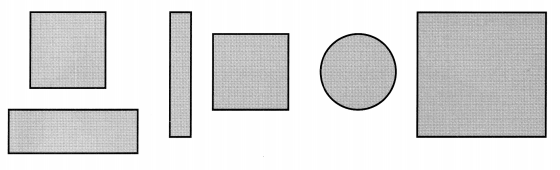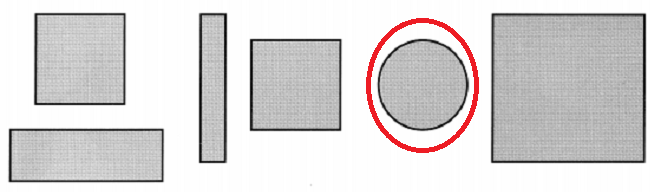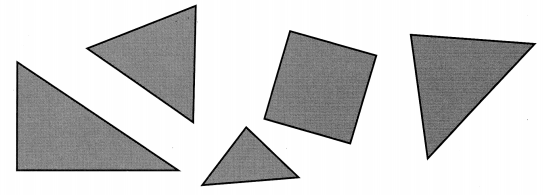# Math in Focus Grade 1 Chapter 5 Practice 1 Answer Key Exploring Plane Shapes

This handy Math in Focus Grade 1 Workbook Answer Key Chapter 5 Practice 1 Exploring Plane Shapes detailed solutions for the textbook questions.

## Math in Focus Grade 1 Chapter 5 Practice 1 Answer Key Exploring Plane Shapes

Trace the dots.

Then match each shape to its name.

Question 1.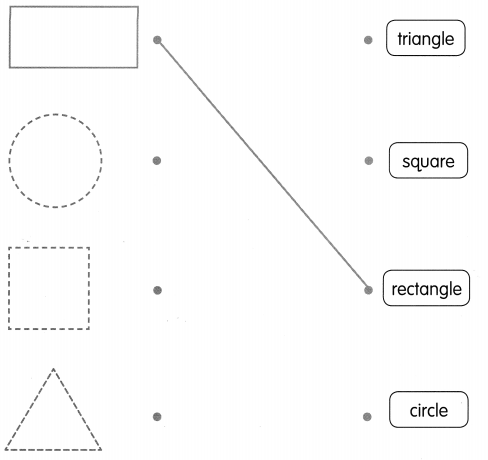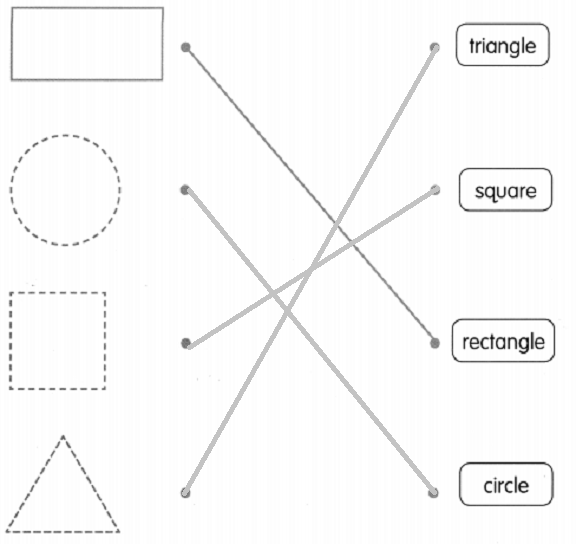A part of each shape is missing. Think about what shape it was. Then match the shape to its name.

Question 2.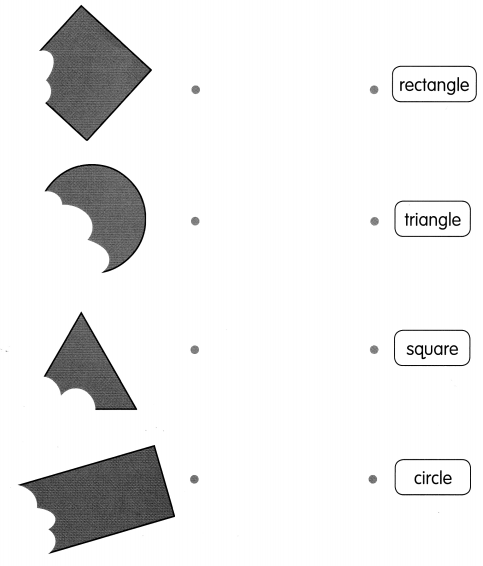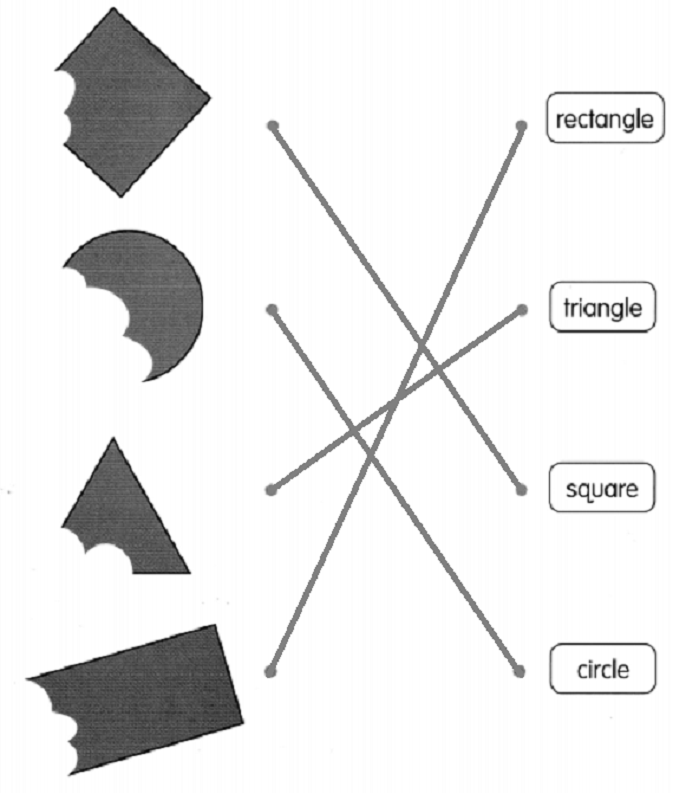Circle the shapes that are the same shape as the shaded shape.

Question 3.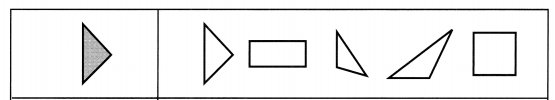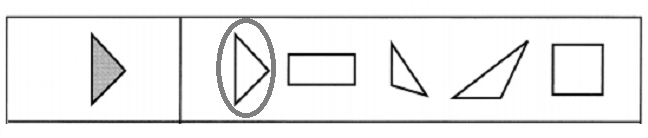Question 4.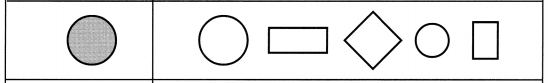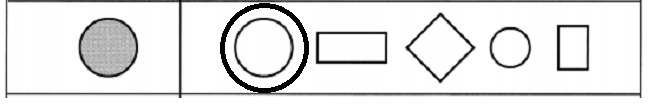Question 5.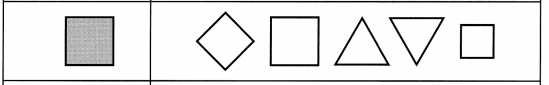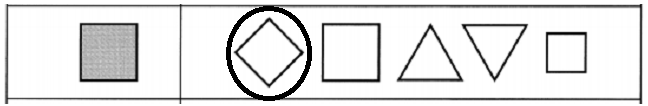Question 6.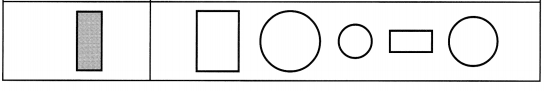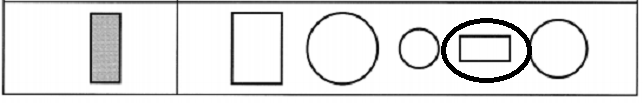Color the shapes.

Question 7.
squares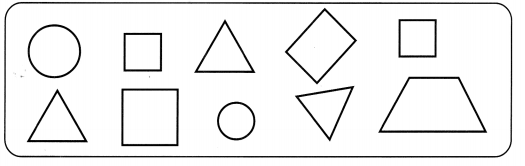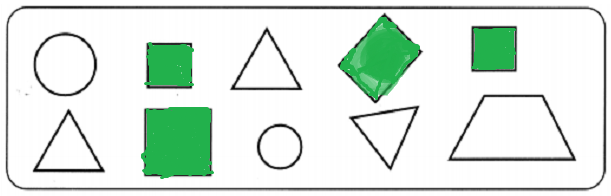Question 8.
triangles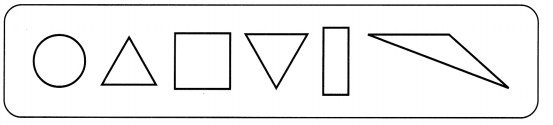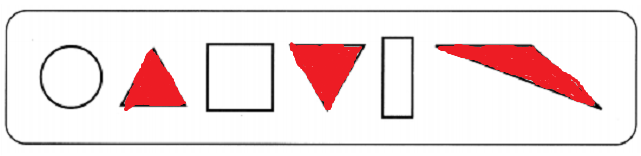Color the shapes.

Question 9.
rectangles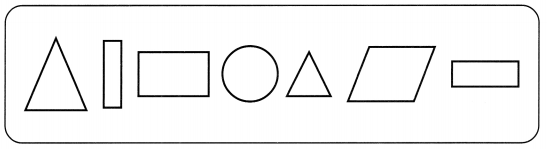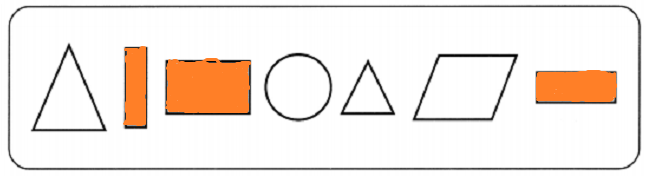Question 10.
The shapes that are not circles.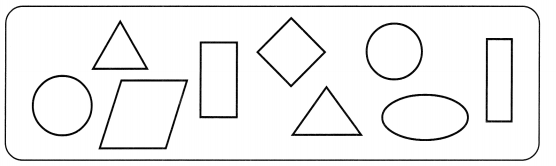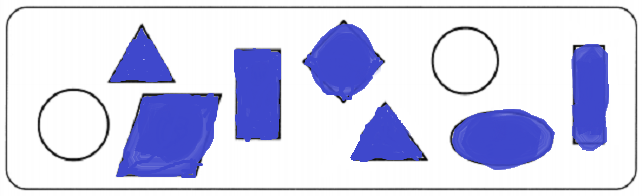Which shape is not in each set? Circle the correct answer.

Question 11.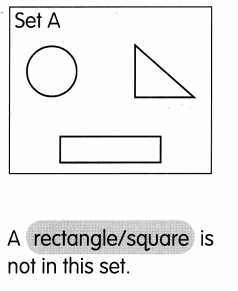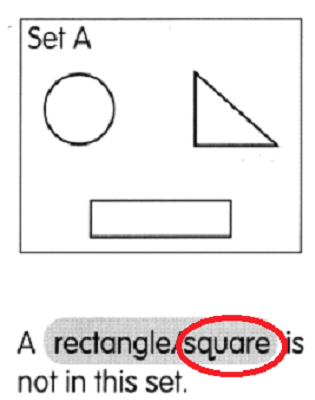A square is not in this set.

Question 12.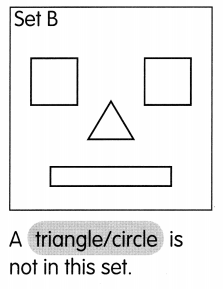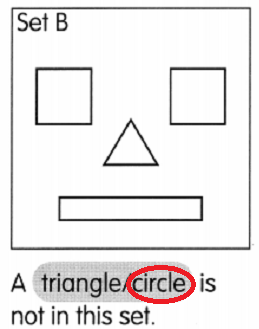A circle is not in this set.

How many sides and corners are there? Count.

Question 13.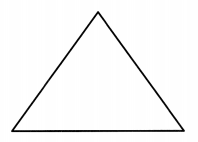___ sides
____ corners
In the above figure there are 3 sides and 3 corners.

Question 14.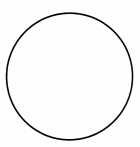___ sides
____ corners
In the above figure there is 0 sides and 0 corners.

Sort the shapes by color.
Circle the shape that is different.

Question 15.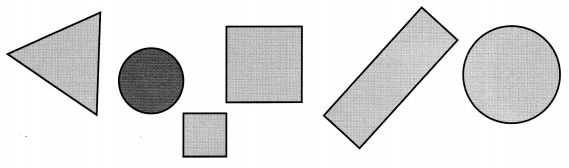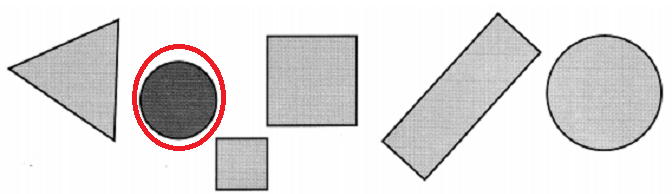Sort the shapes by size.
Color the shapes that are alike.

Question 16.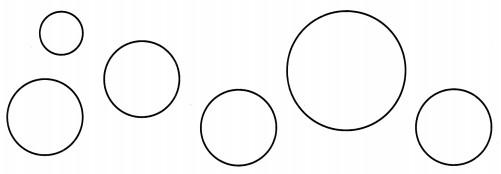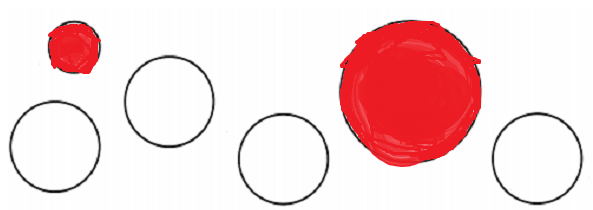Sort the shapes by shape.
Color the shapes that are alike.

Question 17.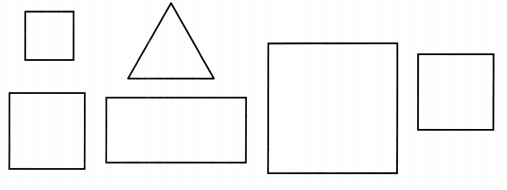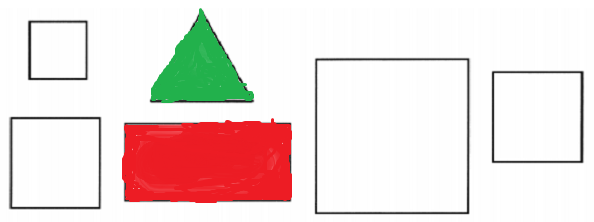Sort the shapes by corners.
Circle the shape that is different.

Question 18.